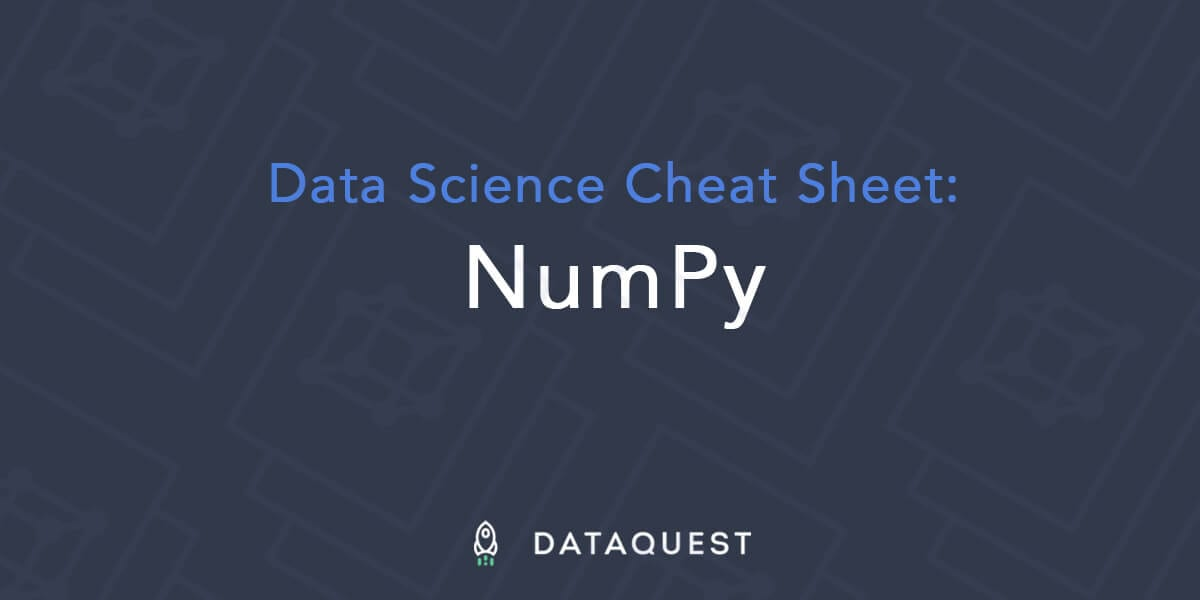# NumPy Cheat Sheet — Python for Data ScienceNumPy is the library that gives Python its ability to work with data at speed. Originally, launched in 1995 as ‘Numeric,’ NumPy is the foundation on which many important Python data science libraries are built, including Pandas, SciPy and scikit-learn.

It’s common when first learning NumPy to have trouble remembering all the functions and methods that you need, and while at Dataquest we advocate getting used to consulting the NumPy documentation, sometimes it’s nice to have a handy reference, so we’ve put together this cheat sheet to help you out!

If you’re interested in learning NumPy, you can consult our NumPy tutorial blog post, or you can signup for free and start learning NumPy through our interactive Python data science course.

Download a Printable PDF of this Cheat Sheet

## Key and Imports

In this cheat sheet, we use the following shorthand:

`arr` | A NumPy Array object

You’ll also need to import numpy to get started:

``import numpy as np``

## Importing/exporting

`np.loadtxt('file.txt')` | From a text file
`np.genfromtxt('file.csv',delimiter=',')` | From a CSV file
`np.savetxt('file.txt',arr,delimiter=' ')` | Writes to a text file
`np.savetxt('file.csv',arr,delimiter=',')` | Writes to a CSV file

## Creating Arrays

`np.array([1,2,3])` | One dimensional array
`np.array([(1,2,3),(4,5,6)])` | Two dimensional array
`np.zeros(3)` | 1D array of length `3` all values `0`
`np.ones((3,4))` | `3`x`4` array with all values `1`
`np.eye(5)` | `5`x`5` array of `0` with `1` on diagonal (Identity matrix)
`np.linspace(0,100,6)` | Array of `6` evenly divided values from `0` to `100`
`np.arange(0,10,3)` | Array of values from `0` to less than `10` with step `3` (eg `[0,3,6,9]`)
`np.full((2,3),8)` | `2`x`3` array with all values `8`
`np.random.rand(4,5)` | `4`x`5` array of random floats between `0``1`
`np.random.rand(6,7)*100` | `6`x`7` array of random floats between `0``100`
`np.random.randint(5,size=(2,3))` | `2`x`3` array with random ints between `0``4`

## Inspecting Properties

`arr.size` | Returns number of elements in `arr`
`arr.shape` | Returns dimensions of `arr` (rows,columns)
`arr.dtype` | Returns type of elements in `arr`
`arr.astype(dtype)` | Convert `arr` elements to type `dtype`
`arr.tolist()` | Convert `arr` to a Python list
`np.info(np.eye)` | View documentation for `np.eye`

## Copying/sorting/reshaping

`np.copy(arr)` | Copies `arr` to new memory
`arr.view(dtype)` | Creates view of `arr` elements with type `dtype`
`arr.sort()` | Sorts `arr`
`arr.sort(axis=0)` | Sorts specific axis of `arr`
`two_d_arr.flatten()` | Flattens 2D array `two_d_arr` to 1D
`arr.T` | Transposes `arr` (rows become columns and vice versa)
`arr.reshape(3,4)` | Reshapes `arr` to `3` rows, `4` columns without changing data
`arr.resize((5,6))` | Changes `arr` shape to `5`x`6` and fills new values with `0`

`np.append(arr,values)` | Appends values to end of `arr`
`np.insert(arr,2,values)` | Inserts values into `arr` before index `2`
`np.delete(arr,3,axis=0)` | Deletes row on index `3` of `arr`
`np.delete(arr,4,axis=1)` | Deletes column on index `4` of `arr`

## Combining/splitting

`np.concatenate((arr1,arr2),axis=0)` | Adds `arr2` as rows to the end of `arr1`
`np.concatenate((arr1,arr2),axis=1)` | Adds `arr2` as columns to end of `arr1`
`np.split(arr,3)` | Splits `arr` into `3` sub-arrays
`np.hsplit(arr,5)` | Splits `arr` horizontally on the `5`th index

## Indexing/slicing/subsetting

`arr` | Returns the element at index `5`
`arr[2,5]` | Returns the 2D array element on index ``
`arr=4` | Assigns array element on index `1` the value `4`
`arr[1,3]=10` | Assigns array element on index `` the value `10`
`arr[0:3]` | Returns the elements at indices `0,1,2` (On a 2D array: returns rows `0,1,2`)
`arr[0:3,4]` | Returns the elements on rows `0,1,2` at column `4`
`arr[:2]` | Returns the elements at indices `0,1` (On a 2D array: returns rows `0,1`)
`arr[:,1]` | Returns the elements at index `1` on all rows
`arr<5` | Returns an array with boolean values
`(arr1<3) & (arr2>5)` | Returns an array with boolean values
`~arr` | Inverts a boolean array
`arr[arr<5]` | Returns array elements smaller than `5`

## Scalar Math

`np.add(arr,1)` | Add `1` to each array element
`np.subtract(arr,2)` | Subtract `2` from each array element
`np.multiply(arr,3)` | Multiply each array element by `3`
`np.divide(arr,4)` | Divide each array element by `4` (returns `np.nan` for division by zero)
`np.power(arr,5)` | Raise each array element to the `5`th power

## Vector Math

`np.add(arr1,arr2)` | Elementwise add `arr2` to `arr1`
`np.subtract(arr1,arr2)` | Elementwise subtract `arr2` from `arr1`
`np.multiply(arr1,arr2)` | Elementwise multiply `arr1` by `arr2`
`np.divide(arr1,arr2)` | Elementwise divide `arr1` by `arr2`
`np.power(arr1,arr2)` | Elementwise raise `arr1` raised to the power of `arr2`
`np.array_equal(arr1,arr2)` | Returns `True` if the arrays have the same elements and shape
`np.sqrt(arr)` | Square root of each element in the array
`np.sin(arr)` | Sine of each element in the array
`np.log(arr)` | Natural log of each element in the array
`np.abs(arr)` | Absolute value of each element in the array
`np.ceil(arr)` | Rounds up to the nearest int
`np.floor(arr)` | Rounds down to the nearest int
`np.round(arr)` | Rounds to the nearest int

## Statistics

`np.mean(arr,axis=0)` | Returns mean along specific axis
`arr.sum()` | Returns sum of `arr`
`arr.min()` | Returns minimum value of `arr`
`arr.max(axis=0)` | Returns maximum value of specific axis
`np.var(arr)` | Returns the variance of array
`np.std(arr,axis=1)` | Returns the standard deviation of specific axis
`arr.corrcoef()` | Returns correlation coefficient of array

## Download a printable version of this cheat sheet

If you’d like to download a printable version of this cheat sheet you can do so below.

Download a Printable PDF of this Cheat Sheet